Custom SearchPressure and Force in Fluid Power Systems

Figure 2-9.Force transmitted through fluid.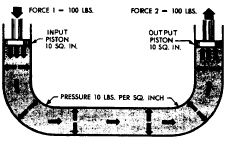Pascal was also the first to prove by experiment that the shape and volume of a container in no way alters pressure. Thus in figure 2-8, if the pressure due to the weight of the liquid at a point on horizontal line H is 8 psi, the pressure is 8 psi everywhere at level H in the system. Equation 2-5 also shows that the pressure is independent of the shape and volume of a container. of this in the system shown in figure 2-9. If there is a resistance on the output piston and the input piston is pushed downward, a pressure is created through the fluid, which acts equally at right angles to surfaces in all parts of the container. If force 1 is 100 pounds and the area of the input piston is 10 square inches, then the pressure in the fluid is 10 psi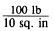Recall that, according to Pascals law, any force applied to a confined fluid is transmitted in all directions throughout the fluid regardless

NOTE: Fluid pressure cannot be created without resistance to flow. In this case, resistanceFigure 2-8.Pressure relationship with shape.

2-6

is provided by the equipment to which the output piston is attached. The force of re-sistance acts against the top of the output piston. The pressure created in the system by the input piston pushes on the underside of the output piston with a force of 10 pounds on each square inch.

In this case, the fluid column has a uniform cross section, so the area of the output piston is the same as the area of the input piston, or 10 square inches. Therefore, the upward force on the output piston is 100 pounds (10 psi x 10 sq. in.), the same as the force applied to the input piston. All that was accomplished in this system was to transmit the 100-pound force around the bend. However, this principle under-lies practically all mechanical applications of fluid power.

At this point you should note that since Pascals law is independent of the shape of the container, it is not necessary that the tube connecting the two pistons have the same cross-sectional area of the pistons. A connection of any size, shape, or length will do, as long as an unobstructed passage is provided. Therefore, the system shown in figure 2-10, with a relatively small, bent pipe connecting two cylinders, will act exactly the same as the system shown in figure 2-9.

MULTIPLICATION OF FORCES. Con-sider the situation in figure 2-11, where the input piston is much smaller than the output piston. Assume that the area of the input piston is 2 square inches. With a resistant force on the output piston a downward force of 20 pounds acting on the input piston creates a pressure of ~ or 10 psi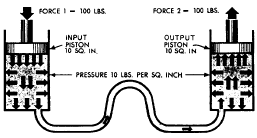Figure 2-10.Transmitting force through a small pipe.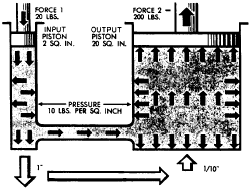Figure 2-11.Multiplication of forces.

in the fluid. Although this force is much smaller than the force applied in figures 2-9 and 2-10, the pressure is the same. This is because the force is applied to a smaller area.

This pressure of 10 psi acts on all parts of the fluid container, including the bottom of the output piston. The upward force on the output piston is 200 pounds (10 pounds of pressure on each square inch). In this case, the original force has been multiplied tenfold while using the same pressure in the fluid as before. In any system with these dimensions, the ratio of output force to input force is always ten to one, regardless of the applied force. For example, if the applied force of the input piston is 50 pounds, the pressure in the system will be 25 psi. This will support a resistant force of 500 pounds on the output piston.

The system works the same in reverse. If we change the applied force and place a 200-pound force on the output piston (fig. 2-11), making it the input piston, the output force on the input piston will be one-tenth the input force, or 20 pounds. (Sometimes such results are desired.) Therefore, if two pistons are used in a fluid power system, the force acting on each piston is directly proportional to its area, and the magnitude of each force is the product of the pressure and the area of each piston.

Note the white arrows at the bottom of figure 2-11 that indicate up and down movement. The movement they represent will be explained later in the discussion of volume and distance factors.

DIFFERENTIAL AREAS. Consider the special situation shown in figure 2-12. Here, a single piston (1) in a cylinder (2) has a piston rod (3) attached to one of its sides. The piston rod extends out of one end of the cylinder. Fluid under pressure is admitted equally to both ends of the cylinder. The opposed faces of the piston (1) behave like two pistons acting against each other. The area of one face is the full cross-sectional area of the cylinder, say 6 square inches, while the area of the other face is the area of the cylinder minus the area of the piston rod, which is 2 square inches. This leaves an effective area of 4 square inches on the right face of the piston. The pressure on both faces is the same, in this case, 20 psi. Applying the rule just stated, the force pushing the piston to the right is its area times the pressure, or 120 pounds (20 x 6). Likewise, the force pushing the piston to the left is its area times the pressure, or 80 pounds (20 x 4). Therefore, there is a net unbalanced force of 40 pounds acting to the right, and the piston will move in that direction. The net effect is the same as if the piston and the cylinder had the same cross-sectional area as the piston rod.

VOLUME AND DISTANCE FACTORS.You have learned that if a force is applied to a system and the cross-sectional areas of the input and output pistons are equal, as in figures 2-9 and 2-10, the force on the input piston will support an equal resistant force on the output piston. The pressure of the liquid at this point is equal to the force applied to the input piston divided by the pistons area. Let us now look at what happens when a force greater than the resistance is applied to the input piston.

In the system illustrated in figure 2-9, assume that the resistance force on the output piston is 100 psi. If a force slightly greater than 100 pounds is applied to the input piston, the pressure in the system will be slightly greater than 10 psi. This increase in pressure will overcome the resistance force on the output piston. Assume that the input piston is forced downward 1 inch. The movement displaces 10 cubic inches of fluid. The fluid must go somewhere. Since the system is closed and the fluid is practically incompressible, the fluid will move to the right side of the system. Because the output piston also has a cross-sectional area of 10 square inches, it will move 1 inch upward to accommodate the 10 cubic inches of fluid. You may generalize this by saying that if two pistons in a closed system have equal cross-sectional areas and one piston is pushed and moved, the other piston will move the same distance, though in the opposite direction. This is because a decrease in volume in one part of the system is balanced by one equal increase in volume in another part of the system.

Apply this reasoning to the system in figure 2-11. If the input piston is pushed down a distance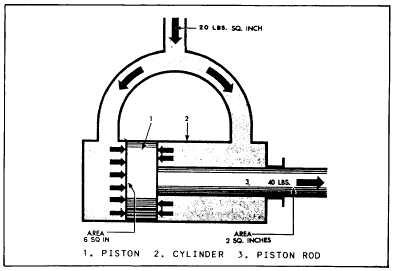Figure 2-12.Differential areas on a piston.

of 1 inch, the volume of fluid in the left cylinder will decrease by 2 cubic inches. At the same time, the volume in the right cylinder will increase by 2 cubic inches. Since the diameter of the right cylinder cannot change, the piston must move upward to allow the volume to increase. The piston will move a distance equal to the volume increase divided by the surface area of the piston (equal to the surface area of the cylinder). In this example, the piston will move one-tenth of an inch (2 cu. in. 20 sq. in.). This leads to the second basic rule for a fluid power system that contains two pistons: The distances the pistons move are inversely proportional to the areas of the pistons. Or more simply, if one piston is smaller than the other, the smaller piston must move a greater distance than the larger piston any time the pistons move.Integrated Publishing, Inc. - A (SDVOSB) Service Disabled Veteran Owned Small Business